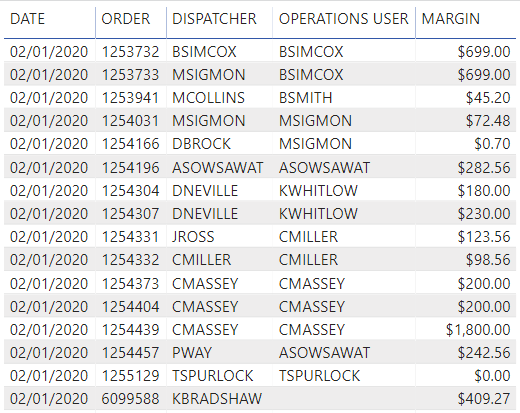cancel
Showing results for
Did you mean:Frequent Visitor

## Calculate the sum of one column based on another column

Hello guys,

I'm having issues with a simple measure. I'd like to SUM column MARGIN by OPERATIONS USER only.

I've tried the following:

a. CALCULATE(SUM('Table'[MARGIN]), ALL('Table' [OPERATIONS USER]))

b. CALCULATE(SUM('Table'[MARGIN]), FILTER('Table', 'Table'[OPERATIONS USER]))

But neither one of the has given me the right results.1 ACCEPTED SOLUTIONCommunity Support

HI @Ma_Betancour,

You can try to use the following measure formula if it meets for your requirement:

``````Measure =
CALCULATE (
SUM ( 'Table'[MARGIN] ),
ALLSELECTED ( 'Table' ),
VALUES ( 'Table'[OPERATIONS USER] )
)``````

If above not help, please share some sample data to test:

Regards,

Xiaoxin Sheng

Community Support Team _ Xiaoxin
If this post helps, please consider accept as solution to help other members find it more quickly.
3 REPLIES 3Anonymous
Not applicable

I have a similar issue. However, in my case, and to use this example, I want to select distinct operations users.Community Support

HI @Ma_Betancour,

You can try to use the following measure formula if it meets for your requirement:

``````Measure =
CALCULATE (
SUM ( 'Table'[MARGIN] ),
ALLSELECTED ( 'Table' ),
VALUES ( 'Table'[OPERATIONS USER] )
)``````

If above not help, please share some sample data to test:

Regards,

Xiaoxin Sheng

Community Support Team _ Xiaoxin
If this post helps, please consider accept as solution to help other members find it more quickly.Impactful Individual

Hi,

Try the below measure:

``CALCULATE(SUM('Table'[MARGIN]), ALLExcept('Table','Table' [OPERATIONS USER])) ``